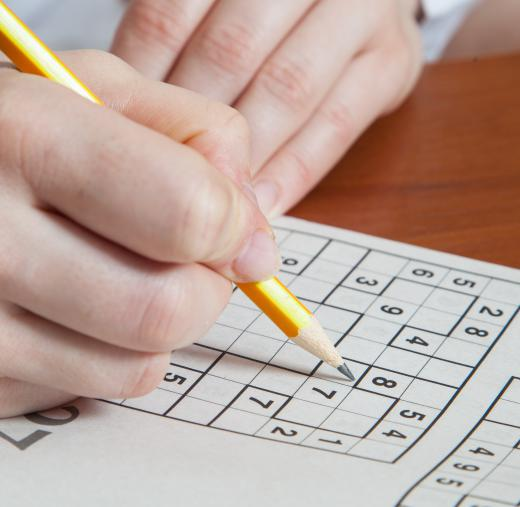# What Are the Different Types of Logic Puzzle Games?

Dee Jones
Dee JonesCrosswords are one form of logic puzzle game.

Logic puzzle games are designed to test an individual’s mental acuity and deductive reasoning skills. Puzzle games can be mini-mysteries, in which the puzzle solver is given a series of facts and asked to find the answer to a question or figure out the solution to a problem. There are many kinds of logic puzzle games, one of the most common being logic riddles, where an individual must use clues, a phrase, or a rhyme to figure out the right answer. Brain teasers can be presented as short stories, strange situations, or mathematical problems, and these puzzles often require a person to think of or see things in a new way to find the right solution. Sudoku puzzles are also popular puzzle games.Sudoku puzzles are a popular kind of logic game.

Logic riddles are popular with both children and adults and can vary in difficulty. In these types of logic puzzle games, an individual is asked to solve a problem or answer a question using the facts found in a question, phrase, or rhyme. One of the most well known logic puzzle riddles has to be, “I’m black and white and re(a)d all over. What am I?” The answer is, “You are a newspaper.” Many logic riddles make use of puns, as in the logic riddle above. When hearing the riddle, a person might assume the final fact in the opening statement is referring to something that is the color red, when it actually refers to something that is “read,” like a book.

Brain teasers are logic puzzle games that also have a strong following. These puzzles can vary in difficulty from quite easy to extremely hard, and can really test and sharpen a person’s mental skills. One well known brain teaser is, “If one man always lies, and one man always tells the truth, what’s the easiest way to determine which is which?” The solution to this brain teaser is simple. Ask both men something with a definite answer, like, “Does two plus two equal four?” The one who always lies will answer, “No,” while the one who always tells the truth will say, “Yes.”

Sudoku puzzles are an extremely popular type of logic puzzle games. In a typical Sudoku puzzle, the puzzle solver is presented with a grid divided into nine rows and nine columns, which are further divided into nine blocks. To solve the puzzle, an individual must place a number from one to nine in each row, column and block without any repeats; so once a three has been placed in a column, another three can’t be placed in the same column. Many find Sudoku puzzles addictive, as well as being a good form of mental exercise.